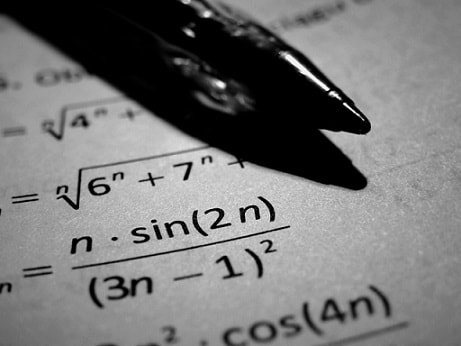# Career in Mathematics

## Introduction to Career in Mathematics

Basic mathematics is essential for every human who lives in this world. It is as an essential tool in many fields includes natural science, engineering, medicine and social science. So the importance of mathematics in day-to-day life is unavoidable. Use of mathematical theory, computational techniques, algorithms, and the latest technology to solve economic, scientific, engineering, physics and business problems. Thus there exists a wide scope for career in mathematics. A person who is an expert in mathematics is called as a mathematician.

## What is Mathematics?

Mathematics deals with study of the measurement, properties and relationships of quantities and sets, using numbers and symbols. The study of mathematics deals with two basic areas: pure mathematics and applied mathematics. Mathematics is widely used to explain phenomena’s and other applications in other fields of study. Some are physics, statistics, engineering, computer science, economics, finance, business, actuarial, chemistry, life sciences, etc.

## Roles and Responsibilities of a Mathematician

Role of mathematician varies with the field in which he / she is working. They will be known in the name of the role and work they are doing instead of mathematician. That is, a mathematician is a business analyst, statistician, operational researcher, medical researcher, engineer in various departments, physics researcher, etc.

## Educational Qualification Required

Basic education required to work in the field of pure mathematics is a B.Sc. degree in pure mathematics. For applied mathematics field the requirement may vary with different fields. A master or post-graduate degree or higher degree like M. Phil or Ph. D in Mathematics is an added advantage for those who like to be in research and academic jobs.

## Skills and Knowledge Required

A mathematician should have good interest in dealing with numbers, problem solving, logical thinking, reasoning skills, concentration, ability to work long time, good communication skills, presentation skills, etc.

The knowledge in theory and its application in various fields is a must for becoming a successful mathematician. Now in the era of computer a mathematician should also possess knowledge of computer programming and software. One should also have knowledge in mathematical modeling and modeling with the use of computer in different fields.

## How to become a Mathematician?

One can become a mathematician by different ways. One option is choosing a science stream with PCM (physics, chemistry and mathematics) as the option and then choosing B.Sc. Mathematics. This person can continue his / her studies to M.Sc. Mathematics, M.Phil. Mathematics or / and Ph.D in Mathematics.

Another way of getting into this fields is through different other means of study which includes applied mathematics, engineering, physics, finance, and various other streams. This is possible because of its wide-spread roots in different fields.

Universities are offering these courses in regular and in distant mode. So a person interested in mathematics can get good option in these fields very easily.

## Where do I get the Job?

A mathematician can get a job in different fields which includes teaching field, statistician, actuary, financial and business analysis, medical research, weather forecasting, business forecasting, operational research, cryptography, banking, engineering, bio-mathematics, etc..